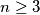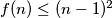MEMO 2009 pojedinačno problem 2

Kvaliteta:
Avg: 4,0
Težina:
Avg: 6,0
Suppose that we have$n \ge 3$ distinct colours. Let$f(n)$ be the greatest integer with the property that every side and every diagonal of a convex polygon with$f(n)$ vertices can be coloured with one of$n$ colours in the following way:

(i) At least two colours are used,

(ii) any three vertices of the polygon determine either three segments of the same colour or of three different colours.

Show that$f(n) \le (n-1)^2$ with equality for infintely many values of$n$.
Izvor: Srednjoeuropska matematička olimpijada 2009, pojedinačno natjecanje, problem 2